# Multiplying Complex Numbers using FOIL

Author: Sophia Tutorial
##### Description:

Multiply complex numbers using FOIL and simplify using the definition of i squared.

(more)

Sophia’s self-paced online courses are a great way to save time and money as you earn credits eligible for transfer to many different colleges and universities.*

No credit card required

37 Sophia partners guarantee credit transfer.

299 Institutions have accepted or given pre-approval for credit transfer.

* The American Council on Education's College Credit Recommendation Service (ACE Credit®) has evaluated and recommended college credit for 33 of Sophia’s online courses. Many different colleges and universities consider ACE CREDIT recommendations in determining the applicability to their course and degree programs.

Tutorial
what's covered
This tutorial covers how to multiply complex numbers, through the definition and discussion of:
1. Imaginary and Complex Numbers
2. Multiplying Imaginary and Complex Numbers
3. Multiplying Complex Numbers using FOIL

## 1. Imaginary and Complex Numbers

To review, the square root of a negative number is non-real, or an imaginary number. The imaginary unit i is defined as the square root of -1.

formula
Imaginary Number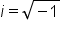If you were to square both sides of this equation, you would have i^2 on the left side, and -1 on the right side, so you also know that i^2 is equal to -1.

formula
Imaginary Number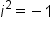A complex number is a value in the form below, in which a and b are real numbers, and i is the imaginary unit.did you know
Complex numbers are used in fields such as engineering and physics.

## 2. Multiplying Imaginary and Complex Numbers

Suppose you want to multiply an imaginary number by a complex number, as in the example: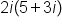You would begin by multiplying these numbers together using distribution, which provides: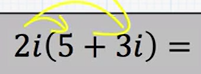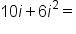Next, you can simplify by remembering that i^2 is equal to -1, so you can rewrite your expression as: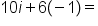Multiplying 6 times -1 gives you the complex number below, which you would rewrite in standard form with the real part first, -6, and the imaginary part second, 10i, to provide your final answer: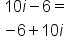## 3. Multiplying Complex Numbers using FOIL

When multiplying complex numbers, use the FOIL method, because of the addition or subtraction that occurs between the real and imaginary parts of complex numbers. Therefore, multiplying complex numbers together is similar to multiplying binomials together.

hint
You may recall that FOIL is an acronym to remember the steps for distributing factors in binomial multiplication:
First
Outside
Inside
Last

Suppose you want to multiply the following complex numbers. Using FOIL, you start by multiplying according to the steps for distributing the factors.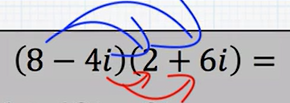Going in order, this provides: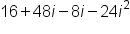Recalling that i^2 is equal to -1, you can substitute -1 in for i^2, then multiply negative 24 times -1.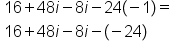To simplify your expression, you know that the real parts are like terms, so you can combine 16 minus -24, which equals 40.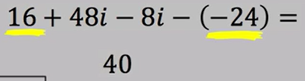You also know that your imaginary parts are like terms so you can combine 48i minus 8i, which equals 40i, so your final answer is 40 plus 40i. Note that this is in standard form.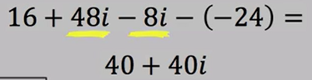summary
Today you reviewed imaginary numbers, recalling that the square root of a negative number is non-real, or an imaginary number; the imaginary unit i is equal to the square root of -1. You also reviewed the definition of a complex number, which is a value in the form a plus bi, where a is the real part and b times i is the imaginary part of the complex number. You learned that when multiplying an imaginary and a complex number together, you use distribution. You also learned that when multiplying two complex numbers together, you use the FOIL method.

Source: This work is adapted from Sophia author Colleen Atakpu.

Formulas to Know
Imaginary NumberRating